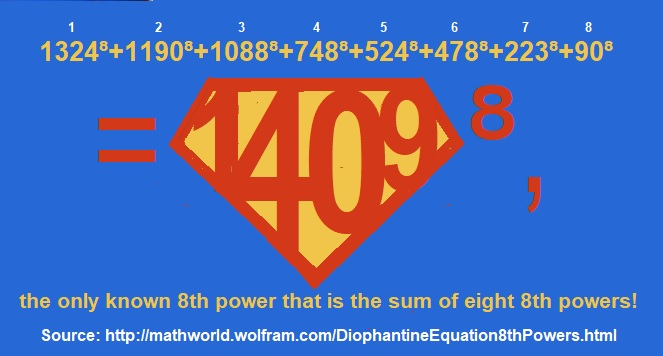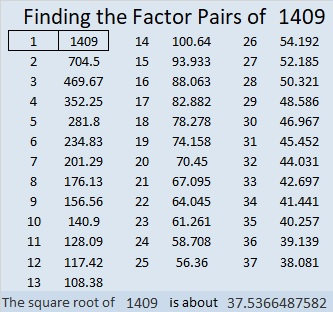# 1409’s Super Power

Stetson.edu informed me that 1409⁸ is the ONLY known 8th power that is the sum of EIGHT 8th powers. Wow! That seems to me to give 1409 quite the superpower!

What were those eight 8th powers that are included in the sum? That’s a puzzle more suited for a computer than a human, but Wolfram Mathworld Diophantine came to my rescue with this POWERFUL fact: 1324⁸+1190⁸+1088⁸+748⁸+524⁸+478⁸+223⁸+90⁸=1409⁸.

Go ahead and check it out on your computer’s calculator. It’s true! Notice also that two of those eighth powers are permutations of each other!

I was so intrigued with 1409 that I had to make this cape so everyone can see how super 1409 is:Sometimes 1409 wears a more modest super cape because 1409² is also the sum of TWO squares:
159² +1400² = 1409²Here are some more super facts about the number 1409:

• 1409 is a prime number.
• Prime factorization: 1409 is prime.
• 1409 has no exponents greater than 1 in its prime factorization, so √1409 cannot be simplified.
• The exponent in the prime factorization is 1. Adding one to that exponent we get (1 + 1) = 2. Therefore 1409 has exactly 2 factors.
• The factors of 1409 are outlined with their factor pair partners in the graphic below.How do we know that 1409 is a prime number? If 1409 were not a prime number, then it would be divisible by at least one prime number less than or equal to √1409. Since 1409 cannot be divided evenly by 2, 3, 5, 7, 11, 13, 17, 19, 23, 29 31, or 37, we know that 1409 is a prime number.

1409 is the sum of two squares:
28² + 25² = 1409

1409 is the hypotenuse of a primitive Pythagorean triple:
159-1400-1409 calculated from 28² – 25², 2(28)(25), 28² + 25²

Here’s another way we know that 1409 is a prime number: Since its last two digits divided by 4 leave a remainder of 1, and 28² + 25² = 1409 with 28 and 25 having no common prime factors, 1409 will be prime unless it is divisible by a prime number Pythagorean triple hypotenuse less than or equal to √1409. Since 1409 is not divisible by 5, 13, 17, 29, or 37, we know that 1409 is a prime number.